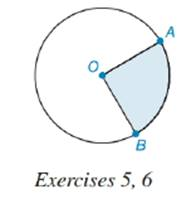Chapter 8.5, Problem 5E### Elementary Geometry for College St...

6th Edition
Daniel C. Alexander + 1 other
ISBN: 9781285195698

#### Solutions

Chapter
Section### Elementary Geometry for College St...

6th Edition
Daniel C. Alexander + 1 other
ISBN: 9781285195698
Textbook Problem
1 views

# In the circle, the radius length is 10 in. and the length of A B ^ is 14 in. What is the perimeter of the shaded sector?To determine

To find:

Explanation

Definition:

Perimeter is the distance around a two dimensional shape.

The distance around the sector is the sum lengths of two radii and the arc intercepted by them.

Formula:

If r is the radius of circle,

Psector=r+r+lintercepted arc=2r+lintercepted arc

Thus, Psector=2r+lintercepted arc

Calculation:

It is given that radius of circle measures 10 in. Thus, r=10.

The arc intercepted by radii OA and OB is AB^

### Still sussing out bartleby?

Check out a sample textbook solution.

See a sample solution

#### The Solution to Your Study Problems

Bartleby provides explanations to thousands of textbook problems written by our experts, many with advanced degrees!

Get Started

#### Expand each expression in Exercises 122. (4+2x)(42x)

Finite Mathematics and Applied Calculus (MindTap Course List)

#### Evaluate the integral. 63. xexdx

Single Variable Calculus: Early Transcendentals

#### f(x) = x3 2x2 + x 1 has a local maximum at: a) 0 b) 1 c) 13 d) f has no local maxima

Study Guide for Stewart's Single Variable Calculus: Early Transcendentals, 8th